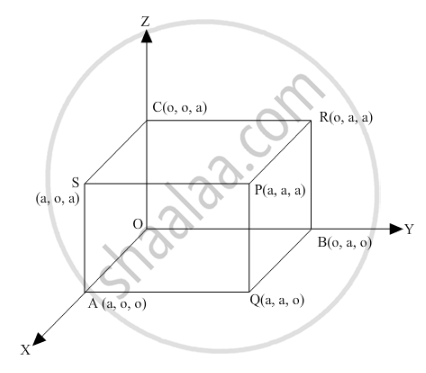Share

The Angle Between the Two Diagonals of a Cube is (A) 30° , (B) 45° ,(C) Cos − 1 ( 1 √ 3 ), (D) Cos − 1 ( 1 3 ). - CBSE (Arts) Class 12 - Mathematics

ConceptDirection Cosines and Direction Ratios of a Line

Question

The angle between the two diagonals of a cube is

• (a) 30°

• (b) 45°

• (c) $\cos^{- 1} \left( \frac{1}{\sqrt{3}} \right)$

• (d) $\cos^{- 1} \left( \frac{1}{3} \right)$

Solution

$\cos^{- 1} \left( \frac{1}{3} \right)$$\text { Let a be the length of an edge of the cube and let one corner be at the origin as shown in the figure . Clearly, OP, AR, BS, and CQ are the diagonals of the cube } .$

$\text{ Consider the diagonals OP and AR } .$

$\text{ Direction ratios of OP and AR are proportional to a - 0, a - 0, a - 0 and 0 - a, a - 0, a - 0, i . e . a, a, a and - a, a, a, respectively } .$

$\text { Let } \theta \text{ be the angle between OP and AR . Then,}$

$\cos \theta = \frac{a \times - a + a \times a + a \times a}{\sqrt{a^2 + a^2 + a^2}\sqrt{\left( - a \right)^2 + a^2 + a^2}}$

$\Rightarrow \cos \theta = \frac{- a^2 + a^2 + a^2}{\sqrt{3 a^2}\sqrt{3 a^2}}$

$\Rightarrow \cos \theta = \frac{1}{3}$

$\Rightarrow \theta = \cos^{- 1} \left( \frac{1}{3} \right)$

$\text{ Similarly, the angles between other pairs of the diagonals are equal to } \cos^{- 1} \left( \frac{1}{3} \right) \text{ as the angle between any two diagonals of a cube is } \cos^{- 1} \left( \frac{1}{3} \right) .$

Is there an error in this question or solution?

Video TutorialsVIEW ALL 

Solution The Angle Between the Two Diagonals of a Cube is (A) 30° , (B) 45° ,(C) Cos − 1 ( 1 √ 3 ), (D) Cos − 1 ( 1 3 ). Concept: Direction Cosines and Direction Ratios of a Line.
S